# An Image is Worth Multiple Words Learning Object Level Concepts using Multi-Concept Prompt Learning

This is my reading note for An Image is Worth Multiple Words: Learning Object Level Concepts using Multi-Concept Prompt Learning. This paper proposes a method to learn embedding of multiple concepts for diffusion model, to this ends, it leverages masking in embed and contrast loss.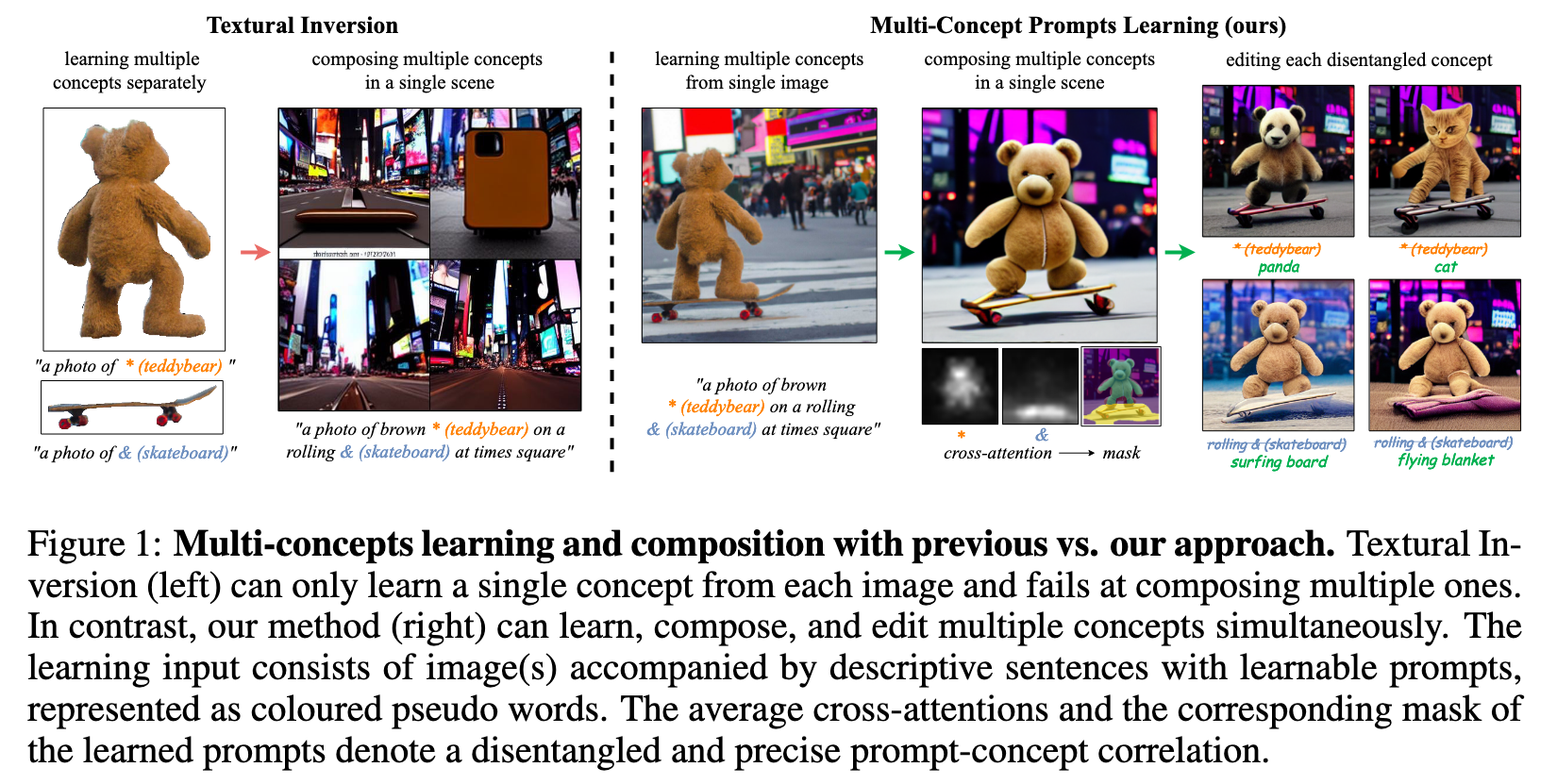# Introduction

Textural Inversion, a prompt learning method, learns a singular embedding for a new “word” to represent image style and appearance, allowing it to be integrated into natural language sentences to generate novel synthesised images. However, identifying and integrating multiple object-level concepts within one scene poses significant challenges even when embeddings for individual concepts are attainable (p. 1)

To address this challenge, we introduce a framework for Multi-Concept Prompt Learning (MCPL), where multiple new “words” are simultaneously learned from a single sentence-image pair. To enhance the accuracy of word-concept correlation, we propose three regularisation techniques: Attention Masking (AttnMask) to concentrate learning on relevant areas; Prompts Contrastive Loss (PromptCL) to separate the embeddings of different concepts; and Bind adjective (Bind adj.) to associate new “words” with known words. (p. 1)

# METHODS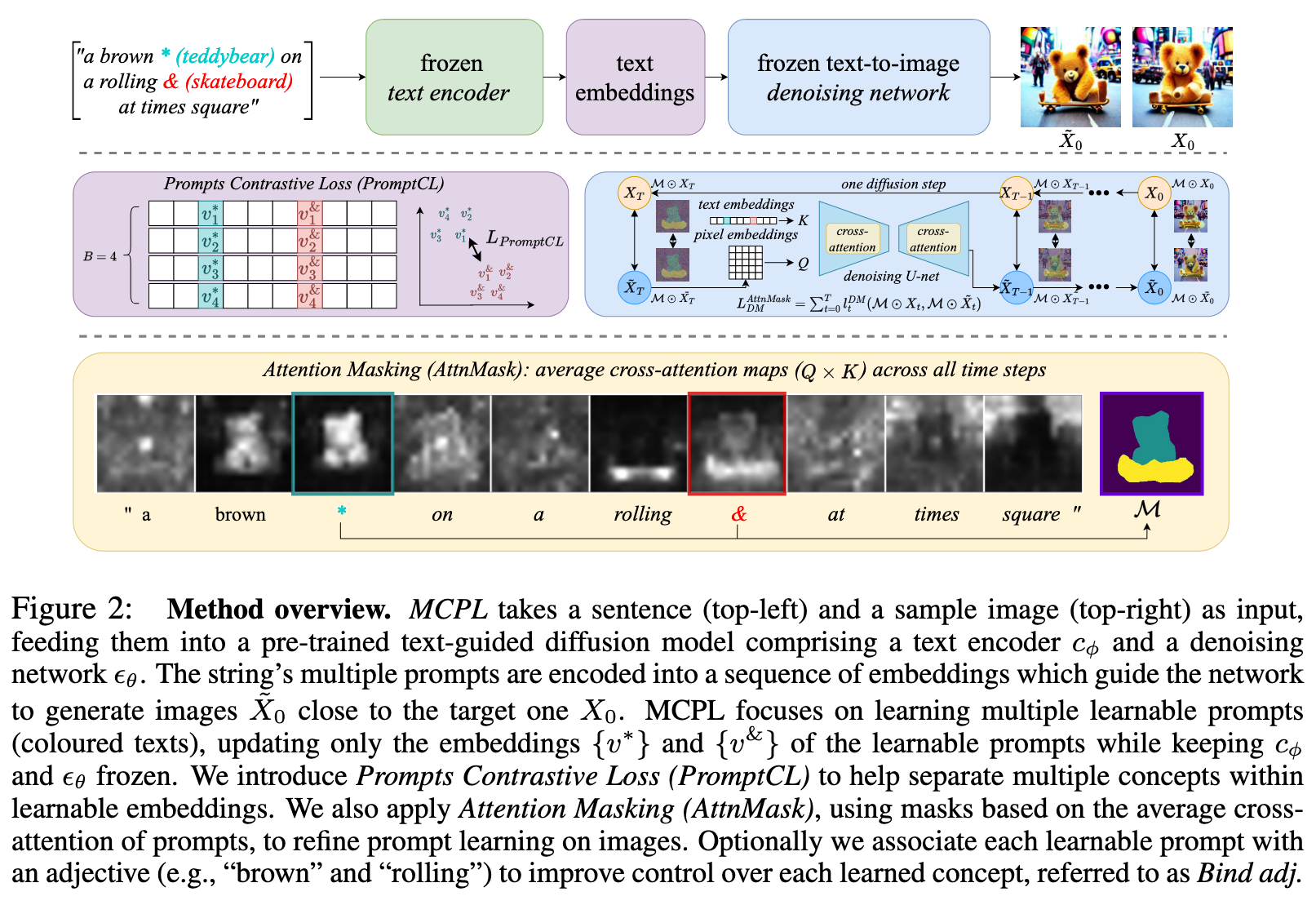## MULTI-CONCEPT PROMPT LEARNING (MCPL)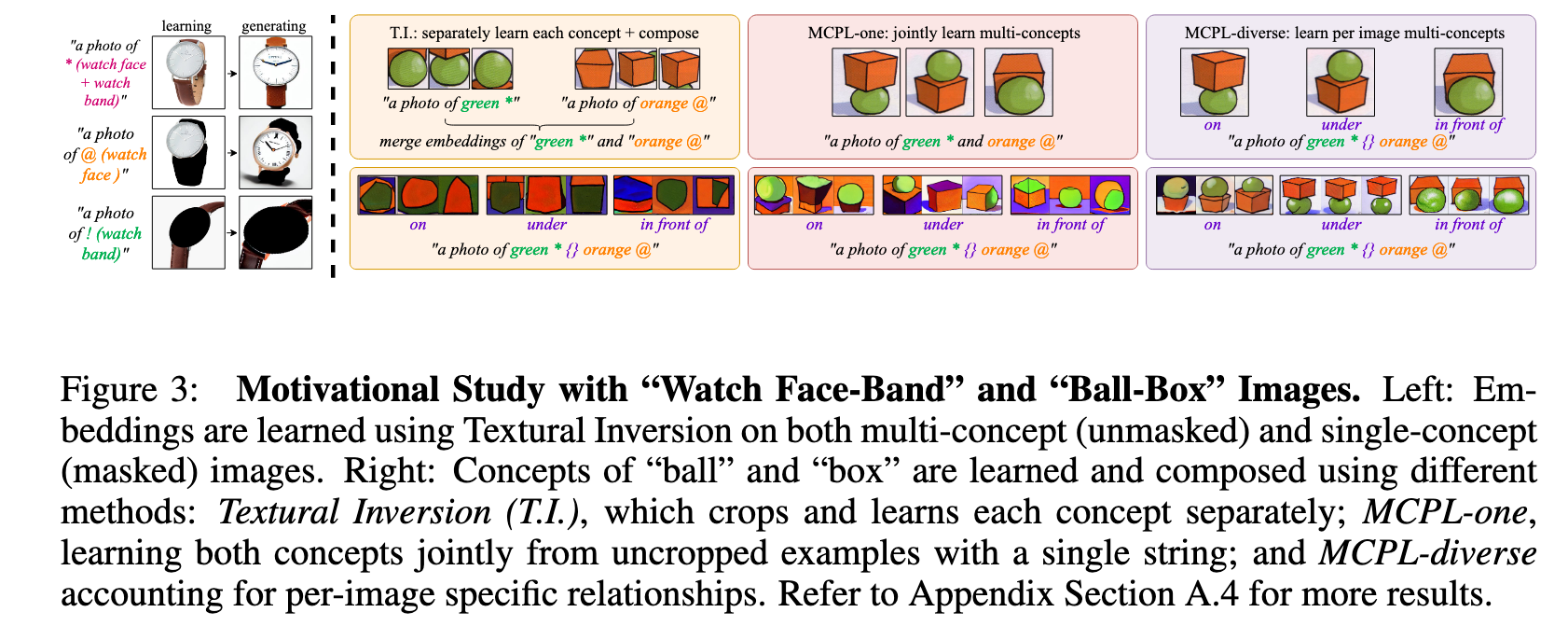Our motivational study confirm that:

1. multiple unique embeddings can be derived from a single multi-concept image, albeit with human intervention,
2. and despite having well-learned individual concepts, synthesizing them into a unified multi-concept scene remains challenging. To address these issues, we introduce the Multi-Concept Prompt Learning (MCPL) framework. MCPL modifies Textural Inversion to enable simultaneous learning of multiple prompts within the same string. In specific, MCPL learn a list of multiple embeddings $V = [v^∗, \cdots , v^&]$ corresponds to multiple new prompts $P = [p^∗, \cdots , p^&]$. The optimisation is still guided by the image-level LDM, but now updating ${v^∗, \cdot , v^&}$ while keeping cϕ and ϵθ frozen (p. 5)

we propose three training strategies: 1) MCPL-all, a naive approach that learns embeddings for all prompts in the string (including adjectives, prepositions and nouns. etc.); 2) MCPL-one, which simplifies the objective by learning single prompt (nouns) per concept; 3) MCPL-diverse, where different strings are learned per image to observe variances among examples. (p. 5)

Our findings indicate that MCPL-one enhance the joint learning of multiple concepts within the same scene over separate learning. Meanwhile, MCPL-diverse goes further by facilitating the learning of intricate relationships between multiple concepts. (p. 5)

### Limitations of plain MCPL

As depicted in Figure 4, both MCPL-one and MCPL-all inadequately capture this correlation, especially for the target concept. These results suggest that naively extending image-level prompt learning techniques (Gal et al., 2022) to object-level multi-concept learning poses optimisation challenges (p. 5)

## REGULARISING THE MULTIPLE OBJECT-LEVEL PROMPTS LEARNING

Previous results show plain MCPL may learn prompts focused on irrelevant areas. To correct this, we apply masks to both generated and target images over all the denoising steps (Figure 2, middle-right). These masks, derived from the average cross-attention of learnable prompts (Figure 2, bottom-row), constrain the image generation loss (equation 1) to focus on pertinent areas, thereby improving prompt-concept correlation. To calculate the mask, we compute for each learnable prompt p ∈ P the average attention map over all time steps Mp = 1/T PT t=1 Mp t . We then apply a threshold to produce binary maps for each learnable prompt, where B(Mp) := {1 if Mp > k, 0 otherwise} and k = 0.5 throughout all our experiments. For multiple prompt learning objectives, the final mask M is a union of multiple binary masks of all learnable prompts M = Sp∈P B(Mp). We compute the Hadamard product of M with x and x˜ to derive our masked loss LAttnMask DM as equation 2. (p. 5)

### Encouraging semantically disentangled multi-concepts with Prompts Contrastive Loss (PromptCL)

Leveraging the mutual exclusivity of multiple objects in a scene, we introduce a contrastive loss in the latent space where embeddings are optimised. Specifically, we employ an InfoNCE loss Oord et al. (2018), a standard in contrastive and representation learning, to encourage disentanglement between groups of embeddings corresponding to distinct learnable concepts (Figure 2, middle-left). (p. 6)

Given our goal is to differentiate the embeddings corresponding to each prompt, we consider the embeddings of the same concept as positive samples while the others as negative (p. 6)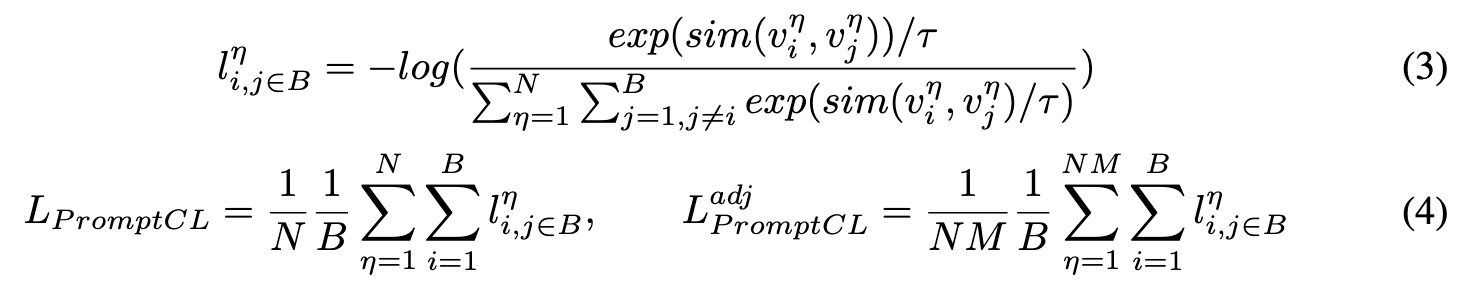### Enhance prompt-concept correlation by binding learnable prompt with the adjective word (Bind adj.)

An additional observation from the misaligned results in Figure 4 reveals that adjective words often correlate strongly with specific regions. This suggests that the pre-trained model is already adept at recognising descriptive concepts like colour or the term ”fluffy.” To leverage this innate understanding, we propose to optionally associate one adjective word for each learnable prompt as one positive group during the contrastive loss calculation (p. 6)

# RESULTS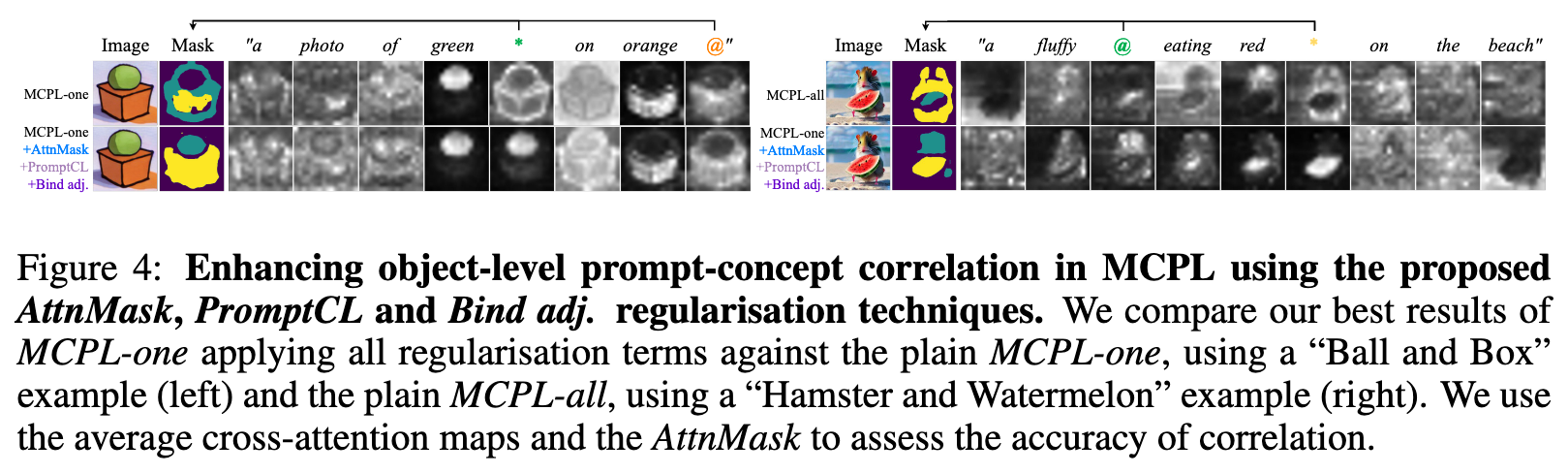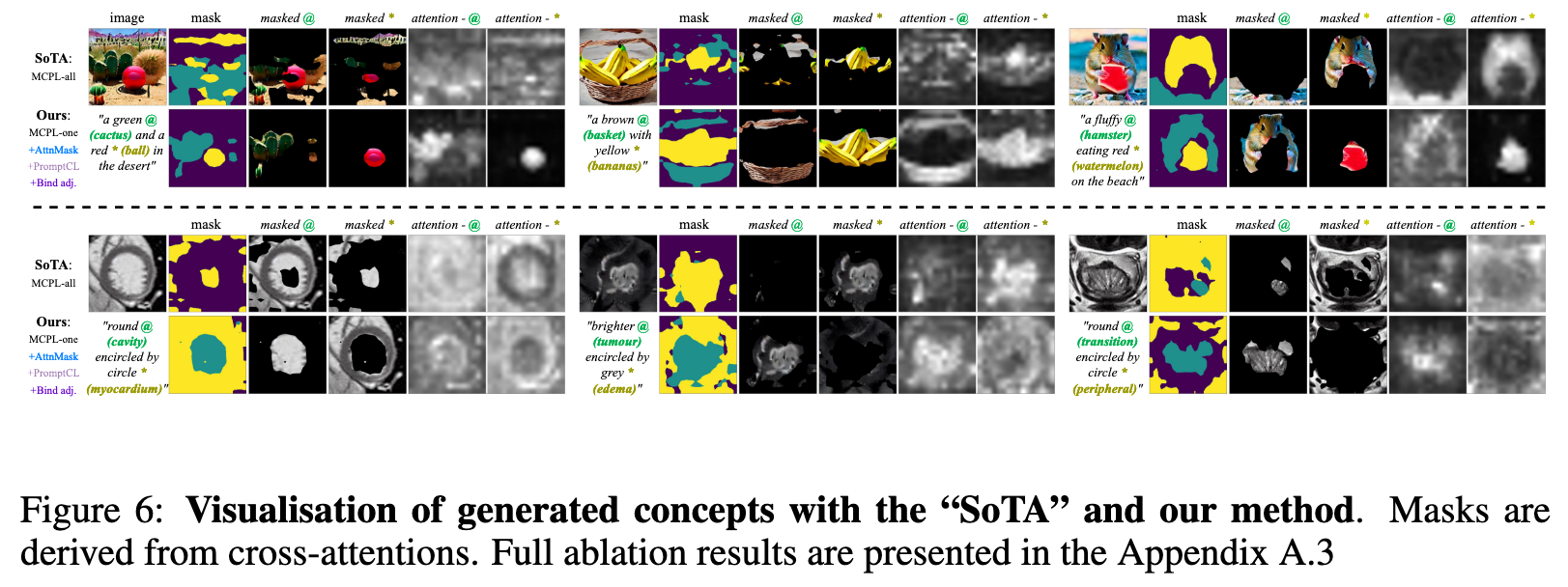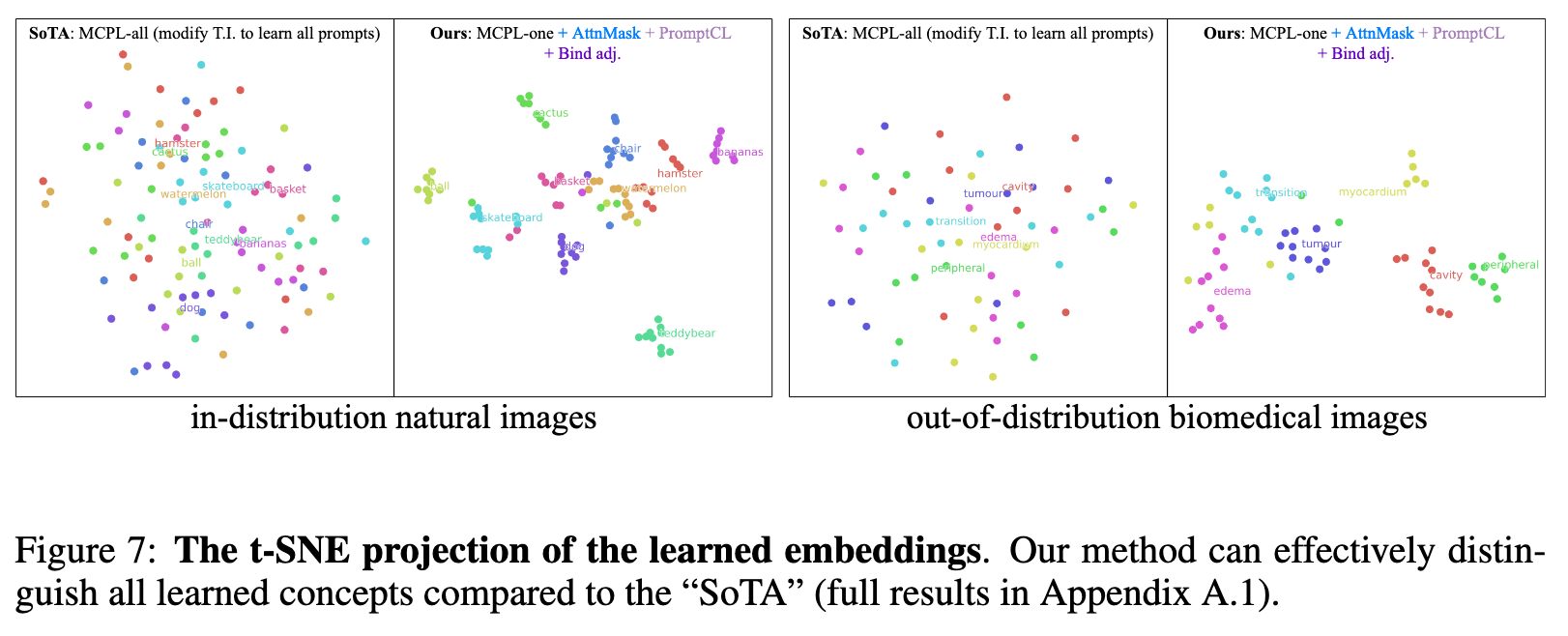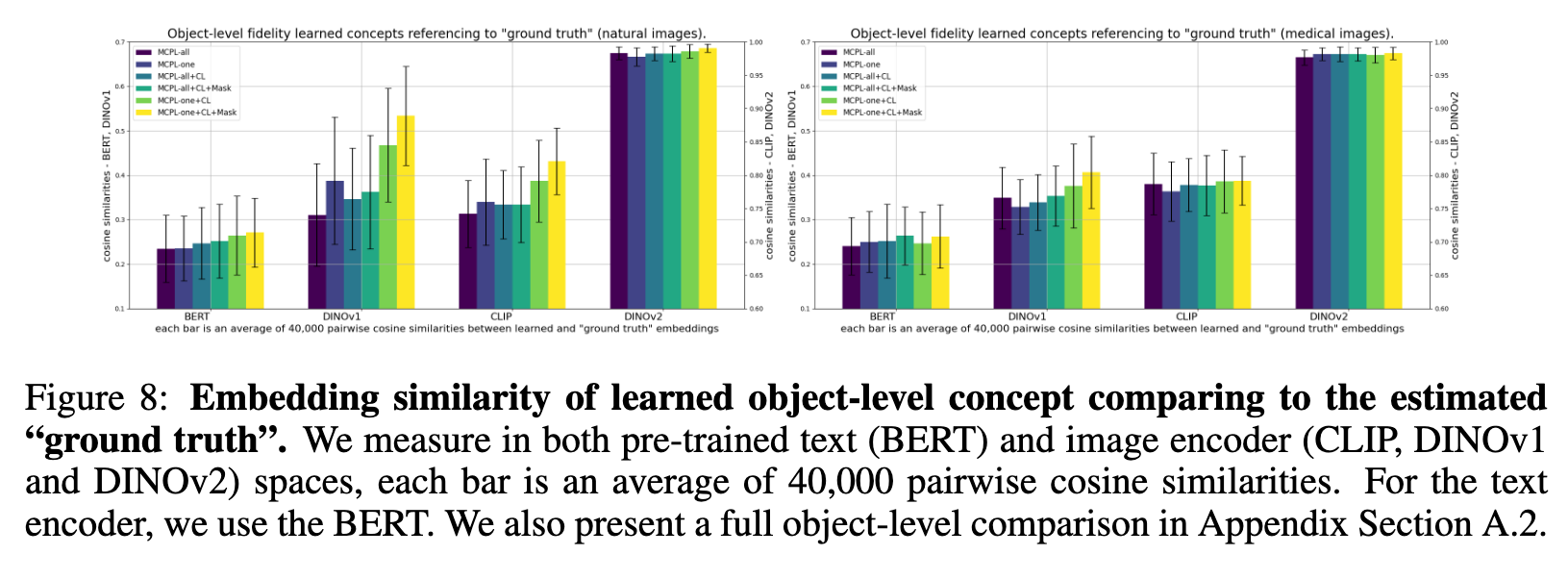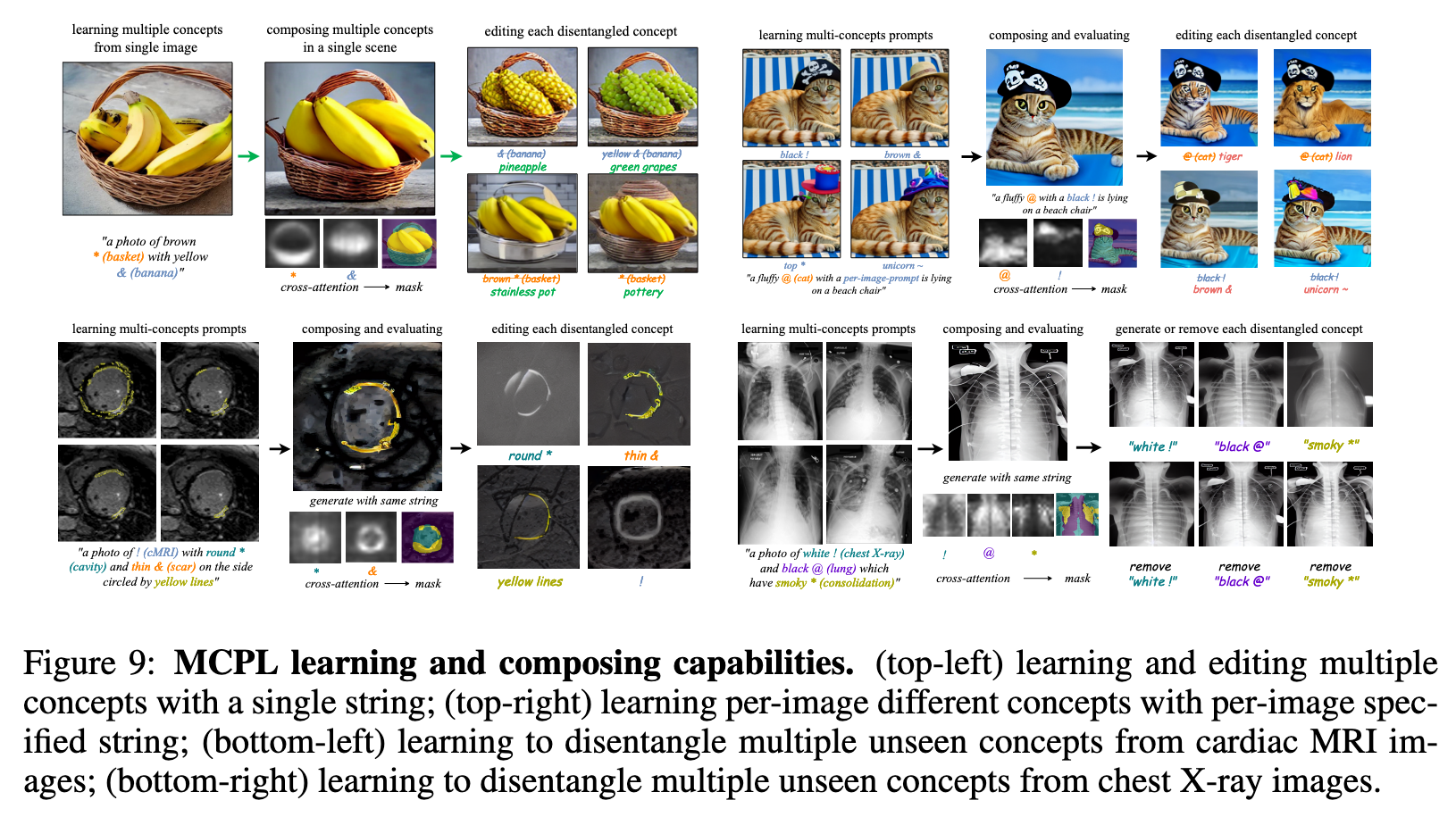# Ablation

Our visual results suggest that incrementally incorporating the proposed regularisation terms enhances concept disentanglement, whereas applying them in isolation yields suboptimal outcomes. Moreover, the results demonstrate that MCPL-one is a more effective learning strategy than MCPL-all, highlighting the importance of excluding irrelevant prompts to maintain a focused learning objective. (p. 20)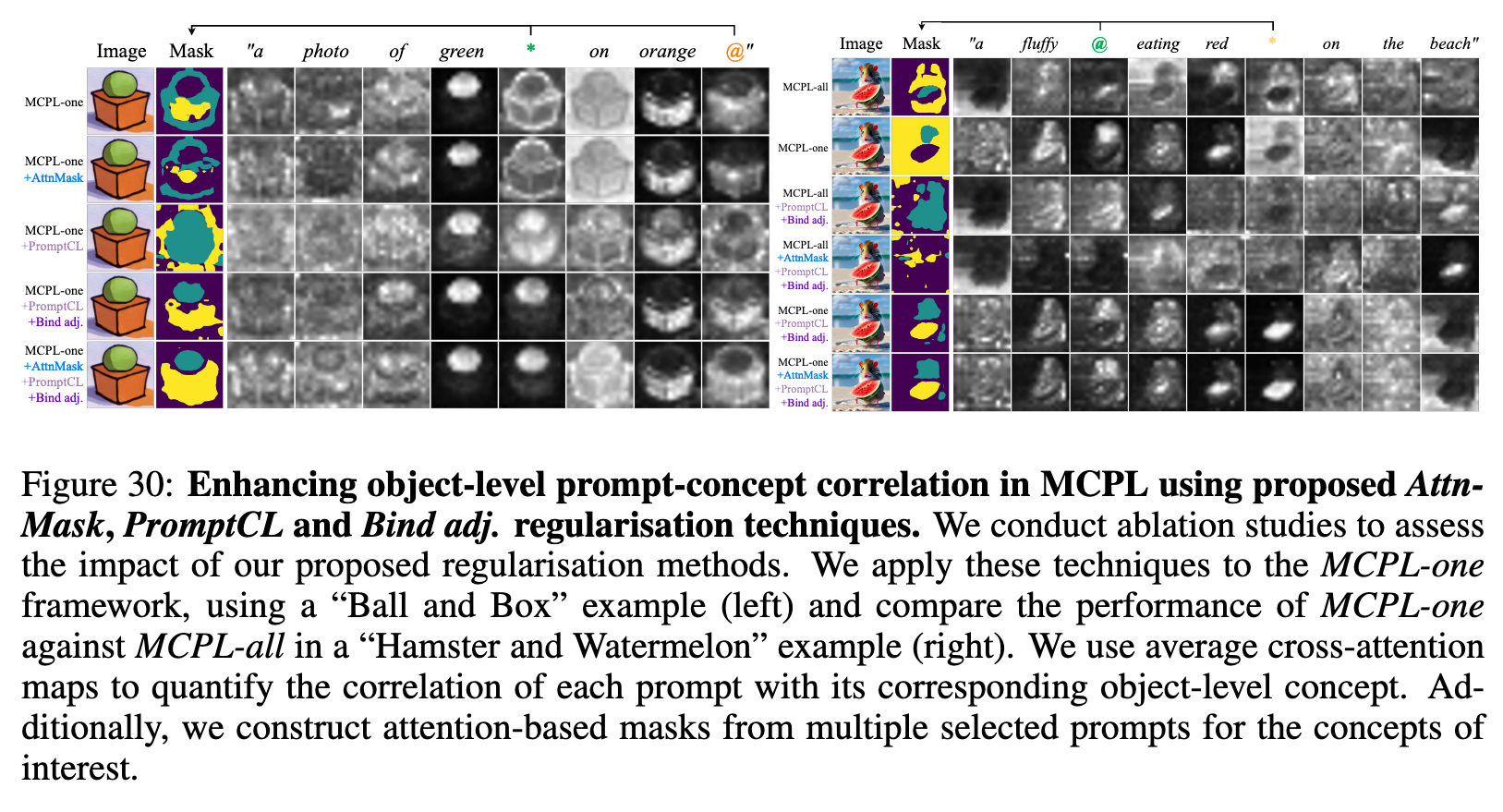Written on June 26, 2023